Homework Help Question & Answers

# 1. Which gas is most dense at 1.00 atm and 23·C? CO, (2 points) .N20, or...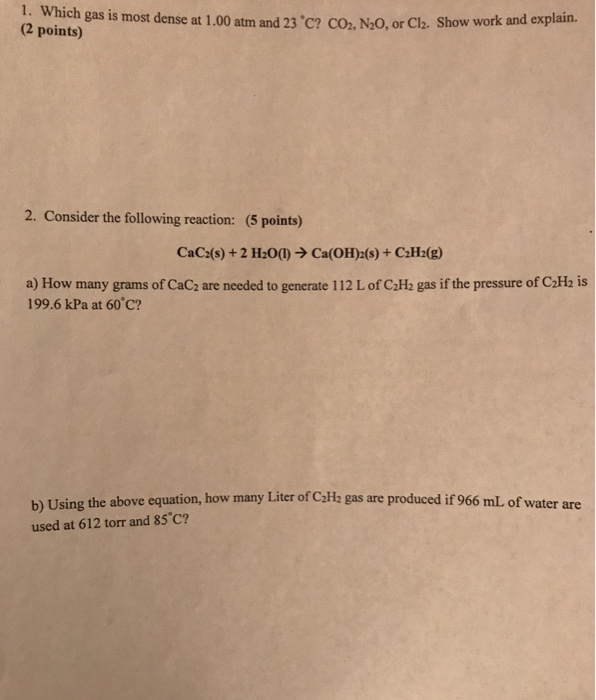1. Which gas is most dense at 1.00 atm and 23·C? CO, (2 points) .N20, or Cla. Show work and explain. 2. Consider the following reaction: (5 points) CaC2(s) + 2 H2O(1) ? Ca(OH)2(s) + C2H2(g) a) How many grams of CaC2 are needed to generate 112 L of C2H2 gas if the pressure of CaHz is 199.6 kPa at 60 c? b) Using the above equation, how many Liter of CzHz gas are produced if 966 mL of water are used at 612 torr and 85°C?

#### Homework Answers

Answer #1

1)

1)

Lets derive the equation to be used
use:
p*V=n*R*T
p*V=(mass/molar mass)*R*T
p*molar mass=(mass/V)*R*T
p*molar mass=density*R*T

So, the one with greater molar mass will be most dense

Molar mass of CO2,
MM = 1*MM(C) + 2*MM(O)
= 1*12.01 + 2*16.0
= 44.01 g/mol

Molar mass of N2O,
MM = 2*MM(N) + 1*MM(O)
= 2*14.01 + 1*16.0
= 44.02 g/mol

Molar mass of Cl2 = 70.9 g/mol

Clearly, molar mass is highest for Cl2

So, Cl2 is most dense

Only 1 question at a time please

Know the answer?
Your Answer:

#### Post as a guest

Your Name:

What's your source?

#### Earn Coin

Coins can be redeemed for fabulous gifts.

Not the answer you're looking for? Ask your own homework help question. Our experts will answer your question WITHIN MINUTES for Free.
Similar Homework Help Questions
• ### A 125 mL glass bulb contains 0.138 g sample of propane, C3H8, at 22C. What is...

A 125 mL glass bulb contains 0.138 g sample of propane, C3H8, at 22C. What is the pressure of this gas? [R = 0.0821 L atm mol-1 K-1] a. 0.61 atm b. 0.28 atm c. 0.045 atm d. 0.0061 atm e. 0.000045 atm Acetylene can be made by reacting calcium carbide with water: CaC2(s) + 2 H2O(l)→Ca(OH)2(s) + C2H2(g) What is the maximum volume of acetylene that can be obtained from 225 g of calcium carbide at 23C and 645...

• ### Hw #3 Acetylene can be produced from calcium carbide and water: Cac,(s)+ 2 H2O(l) → C2H2(g) + Ca(...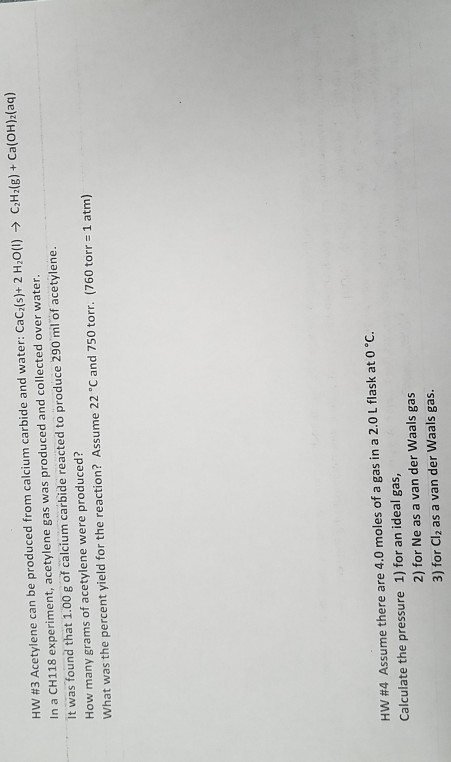Hw #3 Acetylene can be produced from calcium carbide and water: Cac,(s)+ 2 H2O(l) → C2H2(g) + Ca(OH)2(aq) In a CH118 experiment, acetylene gas was produced and collected over water It was found that 1.00 g of calcium carbide reacted to produce 290 ml of acetylene. How many grams of acetylene were produced? What was the percent yield for the reaction? Assume 22 °C and 750 torr. (760 torr 1 atm) HW #4 Assume there are 4.0 moles of a...

• ### show work please 5. (2 points) Calcium carbide reacts with water to produce an unknown gas...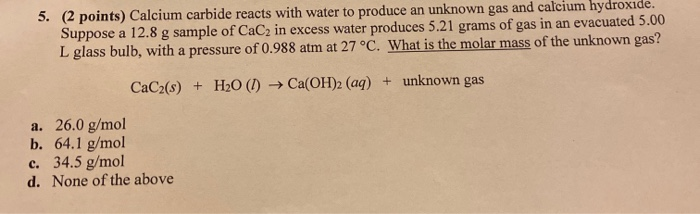show work please 5. (2 points) Calcium carbide reacts with water to produce an unknown gas and calcium hydroxide. Suppose a 12.8 g sample of CaC2 in excess water produces 5.21 grams of gas in an evacuated 5.00 L glass bulb, with a pressure of 0.988 atm at 27 °C. What is the molar mass of the unknown gas? CaC2(s) + H20 (1) Ca(OH)2 (aq) + unknown gas a. 26.0 g/mol b. 64.1 g/mol c. 34.5 g/mol d. None of...

• ### 6. a. Calculate the density of sulfur hexafluoride gas at 709 torr and 23 C. b....

6. a. Calculate the density of sulfur hexafluoride gas at 709 torr and 23 C. b. Calculate the molar mass of a vapor that has a density of 7.125 g/L at 11 ∘C and 745 torr . c. Calcium hydride, CaH2, reacts with water to form hydrogen gas: CaH2(s)+2H2O(l)→Ca(OH)2(aq)+2H2(g) This reaction is sometimes used to inflate life rafts, weather balloons, and the like, where a simple, compact means of generating H2 is desired. How many grams of CaH2 are needed...

• ### Gas pressure

Calcium hydride, CaH2, reacts with water to form hydrogen gas. CaH2(s) + 2 H2O(l) Ca(OH)2(aq) + 2 H2(g) This reaction is sometimes used to inflate life rafts, weatherballoons, and the like, where a simple, compact means of generating H2 is desired. How many grams of CaH2 are needed to generate 16.0 L of H2 gas if the pressure of H2is 750. torr at 25°C? ___ g

• ### 1. (9 points) Balance the following reactions: H3PO4(1) + HCIE) C4H3 + O2 CO2 + H2O...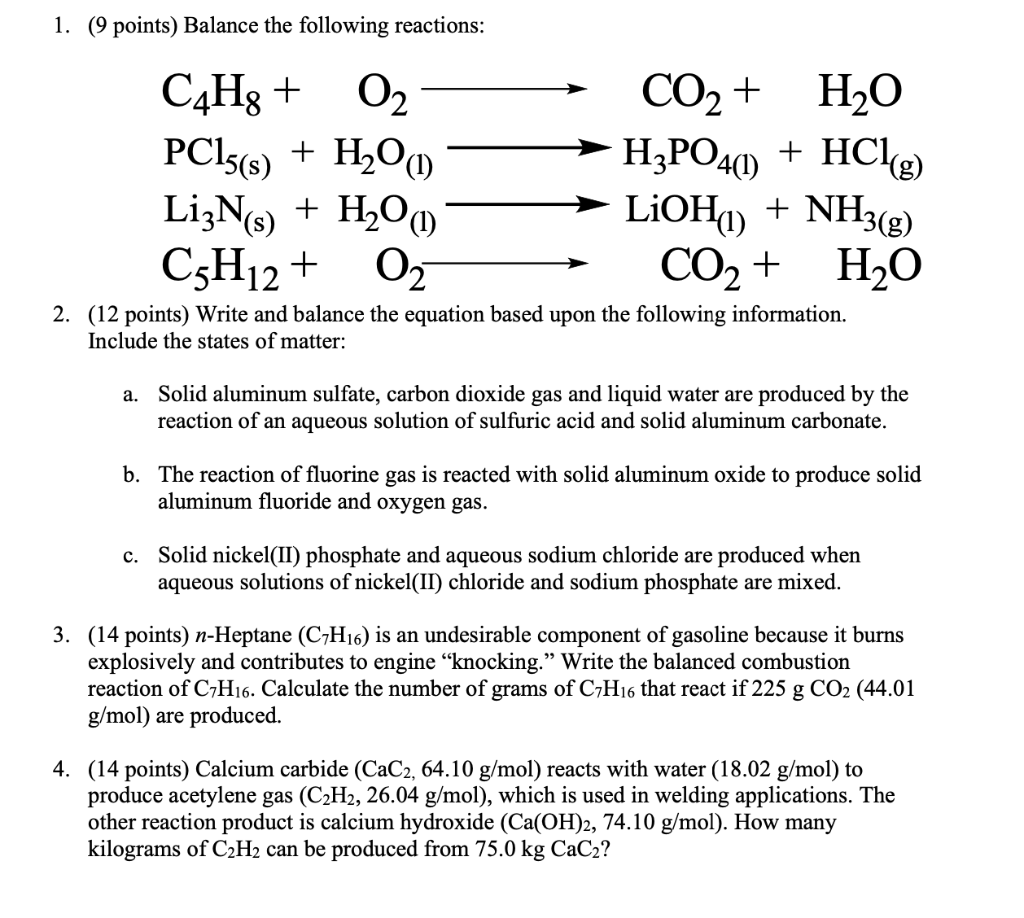1. (9 points) Balance the following reactions: H3PO4(1) + HCIE) C4H3 + O2 CO2 + H2O PC15(s) + H2O LizNs) LiOH) + NH3(g) C3H12 + O2 CO2 + H2O 2. (12 points) Write and balance the equation based upon the following information. + H2O(1) Include the states of matter: a. Solid aluminum sulfate, carbon dioxide gas and liquid water are produced by the reaction of an aqueous solution of sulfuric acid and solid aluminum carbonate. b. The reaction of fluorine...

• ### 3) Calcium hydride, Call, reacts with water to fomm lydrogen gas: CaH2 (s) +2 H2O (l)-Ca(OH)2...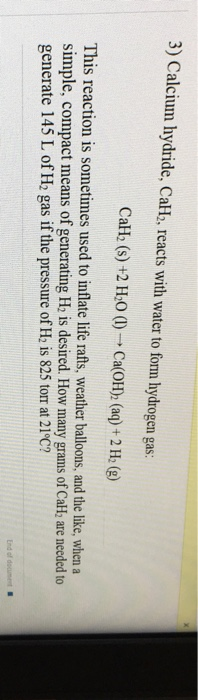3) Calcium hydride, Call, reacts with water to fomm lydrogen gas: CaH2 (s) +2 H2O (l)-Ca(OH)2 (aq) + 2 H2 (g) This reaction is sometimes used to inflate life rafts, weather balloons, and the like, when a simple, compact means of generating H is desired. How many gram generate 145 L of H2 gas if the pressure of He is 825 torr at 21°C? s of CalH are needed to

• ### Calcium hydride, CaH2, reacts with water to form hydrogen gas: CaH2 (s) + H20 (1) -->...

Calcium hydride, CaH2, reacts with water to form hydrogen gas: CaH2 (s) + H20 (1) --> Ca(OH)2 (aq) + 2 H2 (g) This reaction is sometimes used to inflate life rafts when a simple, compact means of generating H2 is desired. How many grams of CaH2 are needed to generate 115 Lof H2 gas with a pressure of 815 torr at 19 °C? (MMCAH2 = 42.098 g/mol, MMH20 = 18.02 g/mol, MMCA(OH)2 = 74.098 g/mol, MMH2 = 2.02 g/mol)

• ### QUESTION 1 A sample of nitrogen gas is sealed into a 18.6 L container at 715.2...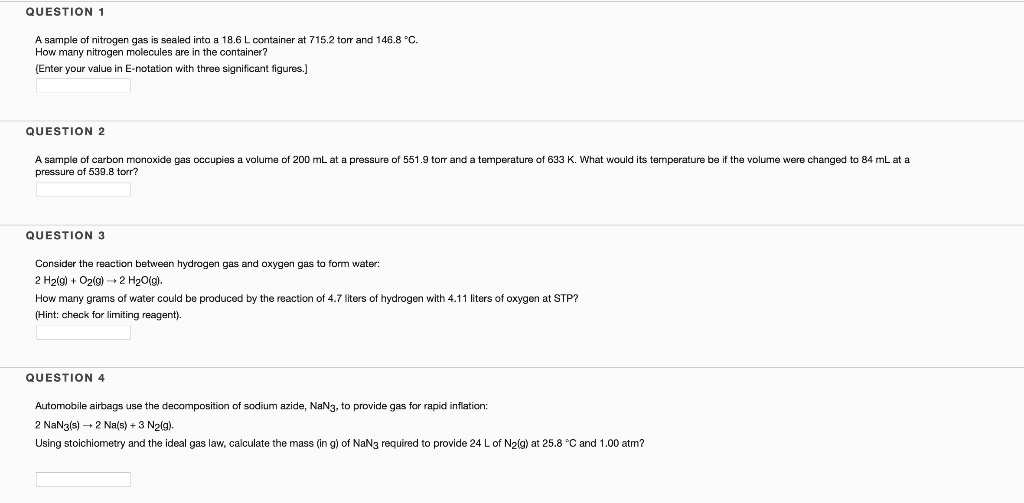QUESTION 1 A sample of nitrogen gas is sealed into a 18.6 L container at 715.2 torr and 146.8 °C. How many nitrogen molecules are in the container? (Enter your value in E-notation with three significant figures.] QUESTION 2 A sample of carbon monoxide gas occupies a volume of 200 mL at a pressure of 551.9 torr and a temperature of 633 K. What would its temperature be if the volume were changed to 84 mL at a pressure of...

• ### - 2. (4 points) Suppose 46.9 grams of chromium(III) oxide and 31.5 grams of silicon are...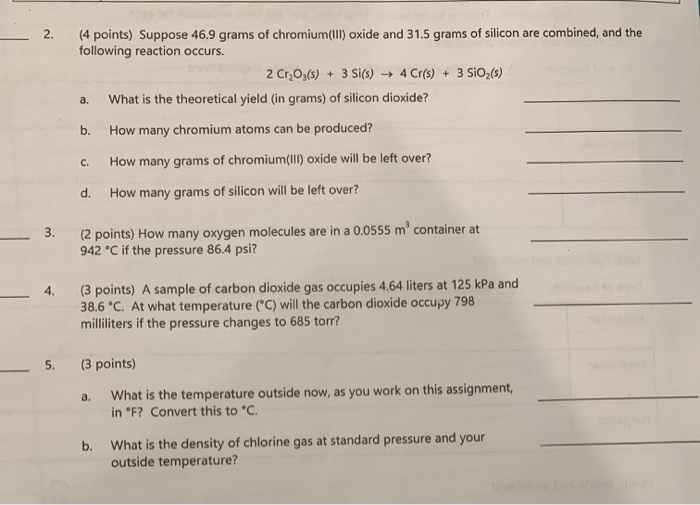- 2. (4 points) Suppose 46.9 grams of chromium(III) oxide and 31.5 grams of silicon are combined, and the following reaction occurs. 2 Cr2O3(s) + 3 Si(s) — 4 Cr(s) + 3 SiO2(5) a. What is the theoretical yield (in grams) of silicon dioxide? b. How many chromium atoms can be produced? C. How many grams of chromium(III) oxide will be left over? d. How many grams of silicon will be left over? 3. (2 points) How many oxygen molecules...

Free Homework App

Scan Your Homework
to Get Instant Free Answers
Need Online Homework Help?

Get Answers For Free
Most questions answered within 3 hours.